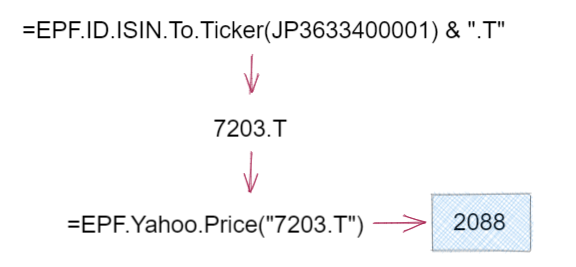# Instrument Identifier Conversion Formulas

Excel Price Feed includes several formulas to help with converting from industry standard financial instrument codes to more commonly used tickers.

These formulas use FIGI (Financial Instrument Global Identifier) to query the mappings between codes and as such are subject to the rate limits set by FIGI, in particular the 25 requests per minute limit; please see FIGI Rate Limits for more details.

We therefore suggest you use these formulas as an intermediate step in constructing your spreadsheet, i.e. use them to find tickers and then afterwards just use the tickers rather than calling the conversion formulas on each spreadsheet refresh.

#### Using the formulas with Yahoo Finance

One of the main motivations for creating these formulas is to enable the Yahoo Finance formulas to be used where the ticker is not known. Please note however that in some cases, particularly non-US stocks, you may need to append the exchange code to the ticker.

For example, the ISIN for Toyota Motor Corp as listed on the Tokyo stock exchange is JP3633400001. Using the conversion formula produces the ticker 7203 however to then use this with the Yahoo Finance formulas the exchange code of .T for Tokyo must be appended. We can then use this ticker to retrieve the current stock price of 2088 JPY:Please see our example spreadsheet which shows how to use each formula as well as how to append the exchange code.

#### Convert from an ISIN to a ticker

An ISIN (International Securities Identification Number) is a 12 digit unique alphanumeric code for identifying a specific security.

The Excel formula used to convert from an ISIN to a ticker is:

`=EPF.ID.ISIN.To.Ticker(ISIN)`

For example, this formula will convert the ISIN US4592001014 to the ticker IBM:

`=EPF.ID.ISIN.To.Ticker(US4592001014)`

#### Convert from a CUSIP to a ticker

A CUSIP (Committee on Uniform Securities Identification Procedures) is a unique identification number assigned to all stocks and bonds in the US and Canada.

The Excel formula used to convert from an CUSIP to a ticker is:

`=EPF.ID.CUSIP.To.Ticker(CUSIP)`

For example, this formula will convert the CUSIP 037833100 to the ticker AAPL:

`=EPF.ID.CUSIP.To.Ticker(037833100)`

#### Convert from a FIGI to a ticker

FIGI (Financial Instrument Global Identifier) is a global standard of the Object Management Group for identifying instruments across all asset classes.

The Excel formula used to convert from a FIGI to a ticker is:

`=EPF.ID.FIGI.To.Ticker(FIGI)`

For example, this formula will convert the FIGI BBG000HW2KR4 to the ticker 7203:

`=EPF.ID.FIGI.To.Ticker(BBG000HW2KR4)`

#### Convert from a SEDOL to a ticker

SEDOL (Stock Exchange Daily Official List) is a seven character UK securities naming system.

The Excel formula used to convert from a SEDOL to a ticker is:

`=EPF.ID.SEDOL.To.Ticker(SEDOL)`

For example, this formula will convert the SEDOL 0540528 to the ticker HSBA:

`=EPF.ID.SEDOL.To.Ticker(0540528)`

#### Convert from a WKN to a ticker

WKN (Wertpapierkennnummer) is a German securities naming system, it is six characters long and contains both alphabetic (excluding the letters I and O), and numeric characters.

The Excel formula used to convert from a WKN to a ticker is:

`=EPF.ID.WKN.To.Ticker(WKN)`

For example, this formula will convert the WKN 519000 to the ticker BMW:

`=EPF.ID.WKN.To.Ticker(519000)`